# Euler's totient function facts for kids

Kids Encyclopedia Facts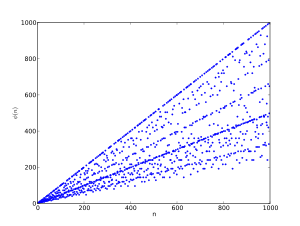The first thousand values of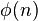$\phi(n)$

In number theory, the totient of a positive integer n is the number of positive integers smaller than n which are coprime to n (they share no factors except 1). It is often written as$\phi (n)$.

For example,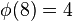$\phi(8) = 4$, because there are four numbers (1, 3, 5 and 7) which do not share any factors with 8. The function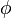$\phi$ used here is the totient function, usually called the Euler totient or Euler's totient, after the Swiss mathematician Leonhard Euler, who studied it. The totient function is also called Euler's phi function or simply the phi function, since the Greek letter Phi ($\phi$) is so commonly used for it. The cototient of n is defined as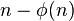$n - \phi(n)$.

The totient function is important mainly because it gives the size of the multiplicative group of integers modulo n. More precisely,$\phi(n)$ is the order of the group of units of the ring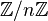$\mathbb{Z}/n\mathbb{Z}$. This fact, together with Lagrange's theorem, provides a proof for Euler's theorem.

A common use of the totient function is in the RSA algorithm. The RSA algorithm is a popular method of encryption used worldwide.

For any prime number, p,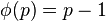$\phi(p) = p-1$.

## Related pagesEuler's totient function Facts for Kids. Kiddle Encyclopedia.